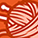cancel
Showing results for
Did you mean:New Contributor III

How are professional researchers doing for avoiding the singularity of Coulomb potential when they do computations about atom or molecules to solve the Schroedinger equation numerically?

Dear ACS Chemists, Sirs!

I am an amature physicist.

I often do computer simulation, or resolve Schroedinger eigenvalue equation using computer.

The problem is the Coulomb potential.

Its amplitude decreases inversely with the distance from a electric charge.

The trouble occurs when I choose a lattice system of which one lattice point coincides with the charge position.

Then the computer shows message that zero division error has occured.

How are the professionals who are doing computation about an actually reallistic atom or a reallistic molecule resolving this trouble in order to obtain a numerically reliable solution?

Sincerely

Pithecantropus Japonicus who, in a cave, is tinkering an abacus without knowing its meaning.

April 15, 2013

3 RepliesNew Contributor II

Re: How are professional researchers doing for avoiding the singularity of Coulomb potential when they do computations about atom or molecules to solve the Schroedinger equation numerically?

Mitsuru,

I can only ask questions since I haven't experienced this type of computer modeling before.

As I understand it, this is a Materials Science and Engineering question more than a physics question.  Are you attempting to describe or predict temporal quantum mechanical behavior of a lattice system with the Schroedinger wave function?  Is there a deviation from Vegard's law? What if you tried separating the temporal and the spacial parts of the equation(s), and fixed each variable to a constant?  Then would you be able to avoid a zero division error and provide a reliable solution?

I hope my response is helpful.

Best Regards,

Deborah Fox WalshNew Contributor III

Re: How are professional researchers doing for avoiding the singularity of Coulomb potential when they do computations about atom or molecules to solve the Schroedinger equation numerically?

Dear Deborah,

Thank you for your response to my inquiry which was directed to the public.

But regrettably, due to my ambiguous use of the word "lattice", you seem to be misunderstanding my question.

Rock crystal or solid solution are irrelevant to my problem.

Usually one should discretize the space coordinates when he tries to solve some differetial equation numerically.  I would mean this discretized space points as the "lattice".

It was my imagination that everybody must understand this word "lattice" as discretized space points.

Let me simplify the core problem.

Deborah, please think that you are going to solve the ground state wave function of a hydrogen atom.

Let us assume that the nucleus, that is, the proton, is fixed at some space point.

Now, how do you address to solve numerically this eigenvalue 3D differential equation?

You must encounter with a big difficulty when you recognize the trouble that the Coulomb potential causes.

I will be looking forward to receiving your response again.

Best regards,

Mitsuru

May 29, 2013New Contributor

Re: How are professional researchers doing for avoiding the singularity of Coulomb potential when they do computations about atom or molecules to solve the Schroedinger equation numerically?

It is quite possible that numerical method has a built in mesh size for the evaluation and the "lattice" constant which you refer to might be an integral multiple of the built in mesh size for numerical evaluation. If you can access the details of your numerical integration/evaluation program and look for what is the smallest interval chosen for the digitization interval (which I referred to as mesh), either you change this in built parameter of the program package or slightly alter your preferred Lattice constant.

Than the overlap of the lattice point with charge position may be not occurring.

Essentially, you do not evaluate at the discontinuity, but the digitized numerical output can be numerically fitted to get an interpolated value at the discontinuity. When you make an interpolation of the fitted analogue function, you do not substitute the charge location (like in actual use of coulomb interaction equation)  explicitly.